Courses

# Routers And Routing Algorithms - MCQ Test - 1

## 20 Questions MCQ Test RRB JE for Computer Science Engineering | Routers And Routing Algorithms - MCQ Test - 1

Description
This mock test of Routers And Routing Algorithms - MCQ Test - 1 for Computer Science Engineering (CSE) helps you for every Computer Science Engineering (CSE) entrance exam. This contains 20 Multiple Choice Questions for Computer Science Engineering (CSE) Routers And Routing Algorithms - MCQ Test - 1 (mcq) to study with solutions a complete question bank. The solved questions answers in this Routers And Routing Algorithms - MCQ Test - 1 quiz give you a good mix of easy questions and tough questions. Computer Science Engineering (CSE) students definitely take this Routers And Routing Algorithms - MCQ Test - 1 exercise for a better result in the exam. You can find other Routers And Routing Algorithms - MCQ Test - 1 extra questions, long questions & short questions for Computer Science Engineering (CSE) on EduRev as well by searching above.
QUESTION: 1

### In the following pairs of OSI protocol layer/sub-layer and its functionality, the INCORRECT pair is

Solution:

Because, Data Link Layer is associated with Frame Synchronization, and not Bit Synchronization.

QUESTION: 2

### Which of the following is NOT true with respect to a transparent bridge and a router?

Solution:

A. Both bridge and router selectively forward data packets => True. Bridge can drop packets not meant for other side, so can router.
B. A bridge uses IP addresses while a router uses MAC addresses => False . A bridge operate at layer 2 (data link layer)
so it uses MAC address, while router at layer 3 (network layer) so it using IP addresse
C. A bridge builds up its routing table by inspecting incoming packets => True. Self Learning Bridges
D. A router can connect between a LAN and a WAN => True. Router connecting home LAN To internet !

QUESTION: 3

### In a network of LANs connected by bridges, packets are sent from one LAN to another through intermediate bridges. Sincemore than one path may exist between two LANs, packets may have to be routed through multiple bridges. Why is thespanning tree algorithm used for bridge-routing?

Solution:

Since, in a spanning tree, there is a unique path from a source to the destination, which avoids loops, since it is a tree, and
contains all the nodes, since it is a spanning tree.

QUESTION: 4

Consider a simple graph with unit edge costs. Each node in the graph represents a router. Each node maintains a routing table indicating the next hop router to be used to relay a packet to its destination and the cost of the path to the destination through that router. Initially, the routing table is empty. The routing table is synchronously updated as follows. In each updation interval, three tasks are performed.
i. A node determines whether its neighbours in the graph are accessible. If so, it sets the tentative cost to each accessible
neighbour as 1. Otherwise, the cost is set to ∞.
ii. From each accessible neighbour, it gets the costs to relay to other nodes via that neighbour (as the next hop).
iii. Each node updates its routing table based on the information received in the previous two steps by choosing the minimum cost.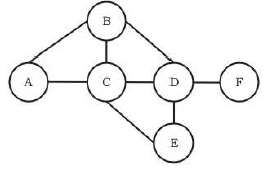For the graph given above, possible routing tables for various nodes after they have stabilized, are shown in the following options. Identify the correct table.

Solution: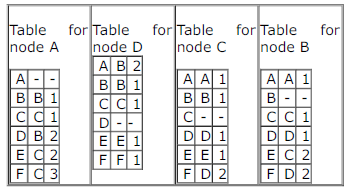correct table are updated only option C is matching here with given tables

QUESTION: 5

Consider a simple graph with unit edge costs. Each node in the graph represents a router. Each node maintains a routing table indicating the next hop router to be used to relay a packet to its destination and the cost of the path to the destination through that router. Initially, the routing table is empty. The routing table is synchronously updated as follows. In each  updation interval, three tasks are performed.
i. A node determines whether its neighbours in the graph are accessible. If so, it sets the tentative cost to each accessible neighbour as 1. Otherwise, the cost is set to ∞.
ii. From each accessible neighbour, it gets the costs to relay to other nodes via that neighbour (as the next hop).
iii. Each node updates its routing table based on the information received in the previous two steps by choosing the minimum cost.Continuing from the earlier problem, suppose at some time t, when the costs have stabilized, node A goes down. The cost from node F to node A at time (t + 100) is :

Solution:

OPTION C
Here, we have to count average hops per message.
Steps -
1) Message goes up from sender to root
2) Message comes down from root to destination

1) Average hops message goes to root -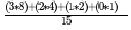= 2.267
Here 3 * 8 represents 3 hops & 8 routers for Bottommost level & So on..

2) Similarly average hops when message comes down -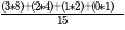{Same as above}
So, Total Hops = 2 * 2.267 = 4.53 (Ans)

QUESTION: 6

A group of 15 routers are interconnected in a centralized complete binary tree with a router at each tree node. Router icommunicates with router j by sending a message to the root of the tree. The root then sends the message back down torouter j. The mean number of hops per message, assuming all possible router pairs are equally likely is

Solution:

The path length differs for nodes from each level. For a node in level 4, we have maximum no. of hops as follows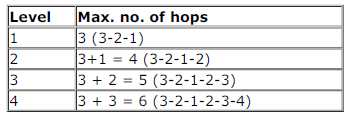So, mean no. of hops for a node in level 4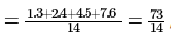as we have 1, 2, 4 and 8 nodes respectively in levels 1, 2, 3 and 4 and we discard the source one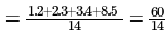in level 4.
Similarly, from a level 3 node we get mean no. of hops,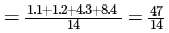From level 2, we get mean no. of hops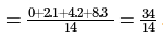And from level 1, we get, mean no. of hops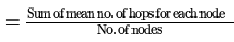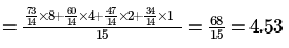So, now we need to find the overall mean no. of hops which will be

QUESTION: 7

A group of 15 routers are interconnected in a centralized complete binary tree with a router at each tree node. Router icommunicates with router j by sending a message to the root of the tree. The root then sends the message back down torouter j. The mean number of hops per message, assuming all possible router pairs are equally likely is

Solution:

The path length differs for nodes from each level. For a node in level 4, we have maximum no. of hops as followsSo, mean no. of hops for a node in level 4as we have 1, 2, 4 and 8 nodes respectively in levels 1, 2, 3 and 4 and we discard the source onein level 4.
Similarly, from a level 3 node we get mean no. of hops,From level 2, we get mean no. of hopsAnd from level 1, we get, mean no. of hopsSo, now we need to find the overall mean no. of hops which will be

QUESTION: 8

Which of the following is TRUE about the interior gateway routing protocols Routing Information Protocol (RIP) and Open Shortest Path First OSPF

Solution:
QUESTION: 9

Consider the following statements about the routing protocols. Routing Information Protocol (RIP) and Oprn Shortest Path
First (OSPF) in an IPv4 network.
I. RIP uses distance vector routing
II. RIP packets are sent using UDP
III. OSPF packets are sent using TCP
IV. OSPF operation is based on link-state routing
Which of the above statements are CORRECT?

Solution:

Statement 1 is CORRECT Bcoz RIP is one of the Oldest DVR(Distance Vector Routing) Protocols which employ the hop count as a routing metric.
Statement 2 is CORRECT Bcoz RIP uses the UDP as its transport protocol with port no 520.
Statement 3 is INCORRECT Bcoz OSPF doesnot use a transport protocol such as UDP or TCP but encapsulates its data directly into IP Packets.
Statement 4 is CORRECT Bcoz OSPF is a routing protocol which uses Link State Routing(LSR) and works within a single Autonomous System.
PS:
OSPF needs to perform reliable multicasting because it needs to talk to multiple possible neighbors on the
same network segment. Now, TCP does not support multicast and UDP is not reliable Therefore, OSPF
implements its own transport mechanism that allows both for reliability (acknowledgements and
retransmissions of lost segments) and multicasting, bypassing both TCP and UDP.
Hence, Option C is CORRECT.

QUESTION: 10

In a RSA cryptosystem, a participant A uses two prime numbers P = 13 and q = 17 to generate here public and private keys. If the public key of A is 35 , then the private key of A is __________ .

Solution: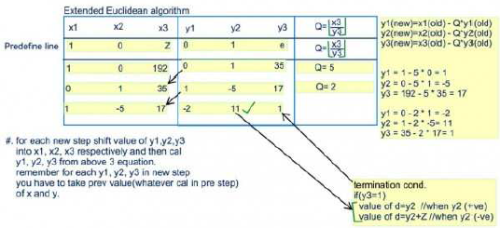Efficient for bigger values

QUESTION: 11

In a RSA cryptosystem, a participant A uses two prime numbers P = 13 and q = 17 to generate here public and private keys. If the public key of A is 35 , then the private key of A is __________ .

Solution:Efficient for bigger values

QUESTION: 12

In a RSA cryptosystem, a participant A uses two prime numbers P = 13 and q = 17 to generate here public and private keys. If the public key of A is 35 , then the private key of A is __________ .

Solution:Efficient for bigger values

QUESTION: 13

Consider a 128 x 103bits/second satellite communication link with one way propagation delay of 150 milliseconds.
Selective retransmission (repeat) protocol is used on this link to send data with a frame size of 1 kilobyte. Neglect the transmission time of acknowledgement. The minimum number of bits required for the sequence number field to achieve 100% utilization is ________.

Solution:

As we want 100 percent efficiency, ws=1+2a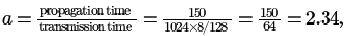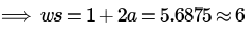Available seq numbers>= ws+wr
In Selective Repeat,
ws=wr(let it be n)
2*n=2*6=12
avail seq numbers>=12
so minimum seq numbers are 12
number of bits for that is ceil of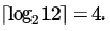QUESTION: 14

Start and stop bits do not contain any "information" but are used in serial communication for

Solution:
QUESTION: 15

In serial communication employing 8 data bits, a parity bit and 2 stop bits, the minimum band rate required to sustain atransfer rate of 300 characters per second is

Solution:

Since stop bit is given it is asynchronous communication and 1 start bit is implied. So,
(8 + 2 + 1 + 1) * 300 = 3600 bps
Minimum band rate required would be 4800 here.

QUESTION: 16

How many bytes of data can be sent in 15 seconds over a serial link with baud rate of 9600 in asynchronous mode with oddparity and two stop bits in the frame?

Solution:

Given that it is asynchronous mode of transmission, then along with per byte, you have to send some extra bit like start,
stop bit and party bits,etc (start and stop bit are compulsory).
1 bit for start bit, 8 bits for data, 1 bit for parity, 2 bits for stop bits.
9600*15/(1+8+1+2) Byte = 12000 Byte.

QUESTION: 17

Count to infinity is a problem associated with

Solution:

Distance vector routing

QUESTION: 18

For the network given in the figure below, the routing tables of the four nodes A, E, D and G are shown. Suppose that F has estimated its delay to its neighbors, A, E, D and G as 8, 10, 12 and 6 msecs respectively and updates its routing table using distance vector routing technique.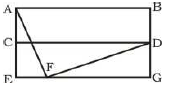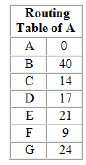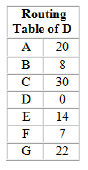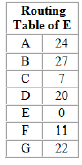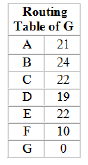Solution:

Distance from F to F is 0 which eliminates option C.
Using distance vector routing protocol, F -> D -> B yields distance as 20 which eliminates option B and D.

QUESTION: 19

Consider the following three statements about link state and distance vector routing protocols, for a large network with 500network nodes and 4000 links.
[S1]: The computational overhead in link state protocols is higher than in distance vector protocols.
[S2]: A distance vector protocol (with split horizon) avoids persistent routing loops, but not a link state protocol.
[S3]: After a topology change, a link state protocol will converge faster than a distance vector protocol.Which one of the following is correct about S1, S2, and S3 ?

Solution:

The computational overhead in link state protocols is higher than in distance vector protocols. Bcz LSR is based upon global knowledge whereas DVR is based upon Local info .
Persistent looping can be avoid with the help of split horizon in DVR.But there is no concept of persisitent looping in LSR,In
LSR only temporary loop exist and can automatically solved by system or router.S2 is false.
And After a topology change, a link state protocol will converge faster than a distance vector protocol.S3 is true.
Option-D

QUESTION: 20

A router uses the following routing table: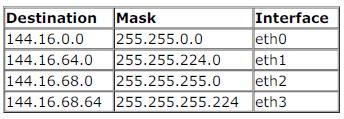packet bearing a destination address 144.16.68.117 arrives at the router. On which interface will it be forwarded?

Solution: